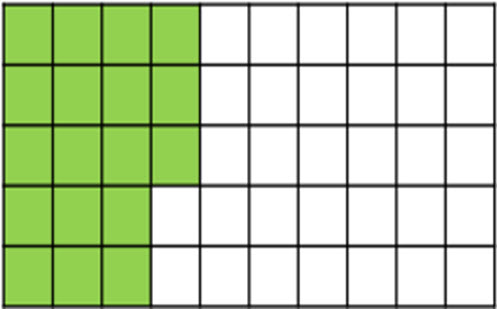# Percentages: understanding, converting and calculating

This guide provides a sequence of small steps for understanding and using percentages, from understanding percentage in terms of quantity and how it relates to fractions and decimals, through to calculating the percentage of an amount.

Year level(s) Year 6
Audience Teacher
Purpose Teaching resource, Teaching strategies
Teaching strategies and pedagogical approaches Questioning, Explicit teaching
Keywords bar model, hundred square, reasoning, problem-solving, explicit teaching

## Curriculum alignment

Curriculum connections Numeracy
Strand and focus Number, Build understanding
Topics Percentage, Decimals, Fractions, Multiplication and division
AC: Mathematics content descriptions
ACMNA131 Make connections between equivalent fractions, decimals and percentages
ACMNA132 Investigate and calculate percentage discounts of 10%, 25% and 50% on sale items, with and without digital technologies
National numeracy learning progression Operating with percentages - OwP1, OwP2, OwP3
Operating with decimals
Interpreting fractions
Multiplicative strategies